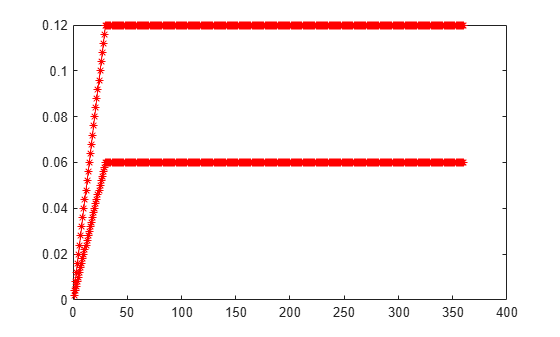# psaspeed2rate

Single monthly mortality rate given PSA speed

## Syntax

``[CPRPSA,SMMPSA] = psaspeed2rate(PSASpeed)``

## Description

example

````[CPRPSA,SMMPSA] = psaspeed2rate(PSASpeed)` calculates vectors of PSA prepayments, each containing 360 prepayment elements, to represent the 360 months in a 30-year mortgage pool.```

## Examples

collapse all

This example shows how to compute the prepayment and mortality rates, given a mortgage-backed security with annual speed set at the PSA default benchmark.

```PSASpeed = [100 200]; [CPRPSA, SMMPSA]= psaspeed2rate(PSASpeed); % view the plot of the output psaspeed2rate(PSASpeed)```## Input Arguments

collapse all

Annual speed relative to the benchmark, specified as any value > `0` using an `NSPD`-by-`1` vector. The PSA benchmark is `100`.

Data Types: `double`

## Output Arguments

collapse all

PSA conditional prepayment rate, returned as a `360`-by-`NSPD` vector in decimals.

PSA monthly default rate, returned as a `360`-by-`NSPD` vector in decimals.

 PSA Uniform Practices, SF-49